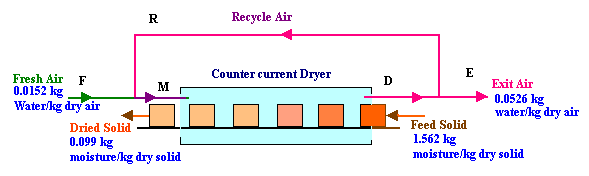### Drying

A stock containing 1.526 kg moisture per kg dry solid is dried to 0.099 kg moisture per kg dry solid by countercurrent air flow. Fresh air entering contains 0.0152 kg water per kg dry sir and the exit air has 0.0526 kg water per kg dry air. What fraction of air is recycled if 52.5 kg of dry air flows per 1 kg of dry solid inside the drier?

Calculations:Basis: 1 kg of dry solid

Material balance for moisture in air:

Water in stream M+ moisture added by drying of solid = Water in stream D

From the problem statement, 52.5 kg dry air is flowing per kg of dry solid and exit air is having a water content of 0.0526 kg per kg of dry air.

Therefore, water in the air leaving the dryer = 52.5 x 0.0526 = 2.7615 kg

Water added by drying of solid = 1.562 - 0.099 = 1.463 kg

Water in the stream M = 2.7615 - 1.463 = 1.2985 kg

Water in stream F + water in stream R = water in stream M

i.e.,

0.0152 F + 0.0526 R = 1.2985   → 1

and balance for dry air:

F + R = 52.5   → 2

Multiplying equation 2 with 0.0152,

0.0152 F + 0.0152 R = 0.798   → 3

subtracting equation 3 from equation 1,

0.0374 R = 0.5005

R = 13.382 kg

Substituting for R in equation 2,

F = 52.5 - 13.382 = 39.118 kg

Recycle ratio = Recycle amount / fresh feed = 13.382 / 39.118 = 0.342